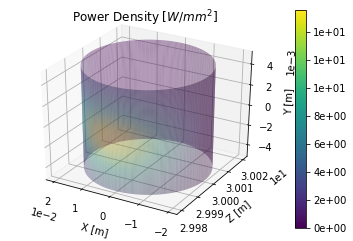# Undulator Power Density on a 3D Object¶

This is a simple example of how to calculate a power density distribution from an undulator on a 3D object.

In :
# Plots inline for notebook
%matplotlib inline

# Import the OSCARS SR module
import oscars.sr

# Import the 3D and parametric surfaces utilities
from oscars.plots3d_mpl import *
from oscars.parametric_surfaces import *

OSCARS v2.1.8 - Open Source Code for Advanced Radiation Simulation
Brookhaven National Laboratory, Upton NY, USA
http://oscars.bnl.gov
oscars@bnl.gov

In :
# Create a new OSCARS object.  Default to 8 threads and always use the GPU if available


## Undulator Field¶

Create a simple undulator field

In :
# Clear all existing fields and create an undulator field
osr.clear_bfields()
osr.add_bfield_undulator(bfield=[0, 1, 0], period=[0, 0, 0.050], nperiods=31)


## Particle Beam and Trajectory¶

Define a particle beam, in this case a 3 [GeV] electron beam. You must also define the start and stop times for the calculation.

In :
# Define simple electron beam
osr.set_particle_beam(energy_GeV=3, x0=[0, 0, -1], current=0.5)

# Define the start and stop times for the calculation
osr.set_ctstartstop(0, 2)


## Create 3D Object and Calculate Power Density¶

Calculate the spectrum 30 [m] downstream from the center of the device on a cylindrical surface.

In :
# First create the surface of interest
cylinder = PSCylinder(R=0.020, L=0.010, nu=101, nv=101)

# Run calculation and plotting
pd = power_density_3d(osr, cylinder, rotations=[osr.pi()/2, 0, 0], translation=[0, 0, 30])